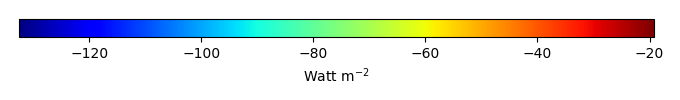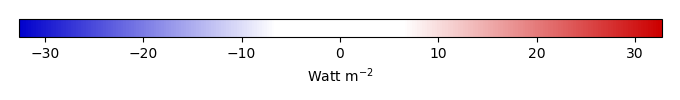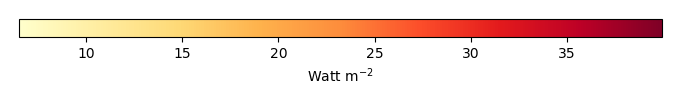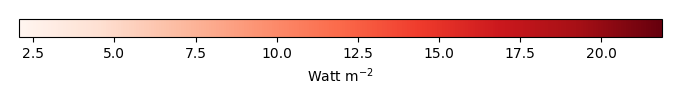# Mean State

Period Mean (original grids) [Watt m-2]
Model Period Mean (intersection) [Watt m-2]
Model Period Mean (complement) [Watt m-2]
Benchmark Period Mean (intersection) [Watt m-2]
Benchmark Period Mean (complement) [Watt m-2]
Bias [Watt m-2]
RMSE [Watt m-2]
Phase Shift [months]
Bias Score 
RMSE Score 
Seasonal Cycle Score 
Spatial Distribution Score 
Interannual Variability Score 
Overall Score 
Benchmark [-] -56.8
CLM4 [-] -61.2 -61.0 -57.0 -52.3 -4.14 13.3 1.69 0.631 0.461 0.756 0.993 0.813 0.686
CLM4.5 [-] -65.3 -65.5 -57.0 -52.3 -8.33 16.2 1.70 0.501 0.458 0.755 0.986 0.828 0.664
CLM5 [-] -66.5 -66.5 -57.0 -52.3 -9.56 16.5 1.67 0.441 0.458 0.762 0.975 0.822 0.652
Period Mean (original grids) [Watt m-2]
Model Period Mean (intersection) [Watt m-2]
Model Period Mean (complement) [Watt m-2]
Benchmark Period Mean (intersection) [Watt m-2]
Benchmark Period Mean (complement) [Watt m-2]
Bias [Watt m-2]
RMSE [Watt m-2]
Phase Shift [months]
Bias Score 
RMSE Score 
Seasonal Cycle Score 
Spatial Distribution Score 
Interannual Variability Score 
Overall Score 
Benchmark [-] -87.0
CLM4 [-] -90.3 -91.1 -87.4 -58.4 -3.32 18.8 0.274 0.777 0.593 0.975 0.948 0.738 0.770
CLM4.5 [-] -95.9 -96.5 -87.4 -58.4 -8.68 21.6 0.279 0.680 0.599 0.975 0.748 0.750 0.725
CLM5 [-] -97.3 -98.0 -87.4 -58.4 -10.2 22.1 0.309 0.676 0.590 0.971 0.811 0.720 0.726
Period Mean (original grids) [Watt m-2]
Model Period Mean (intersection) [Watt m-2]
Model Period Mean (complement) [Watt m-2]
Benchmark Period Mean (intersection) [Watt m-2]
Benchmark Period Mean (complement) [Watt m-2]
Bias [Watt m-2]
RMSE [Watt m-2]
Phase Shift [months]
Bias Score 
RMSE Score 
Seasonal Cycle Score 
Spatial Distribution Score 
Interannual Variability Score 
Overall Score 
Benchmark [-] -81.4
CLM4 [-] -86.5 -86.6 -82.3 -62.6 -2.69 17.2 0.810 0.695 0.523 0.907 0.991 0.730 0.728
CLM4.5 [-] -92.8 -92.9 -82.3 -62.6 -8.33 20.0 0.761 0.594 0.520 0.913 0.973 0.737 0.709
CLM5 [-] -91.9 -92.0 -82.3 -62.6 -7.58 19.7 0.810 0.617 0.506 0.901 0.972 0.712 0.702
Period Mean (original grids) [Watt m-2]
Model Period Mean (intersection) [Watt m-2]
Model Period Mean (complement) [Watt m-2]
Benchmark Period Mean (intersection) [Watt m-2]
Benchmark Period Mean (complement) [Watt m-2]
Bias [Watt m-2]
RMSE [Watt m-2]
Phase Shift [months]
Bias Score 
RMSE Score 
Seasonal Cycle Score 
Spatial Distribution Score 
Interannual Variability Score 
Overall Score 
Benchmark [-] -37.2
CLM4 [-] -37.6 -37.7 -36.9 -41.0 -1.32 15.3 1.10 0.755 0.424 0.862 0.993 0.865 0.720
CLM4.5 [-] -37.6 -37.7 -36.9 -41.0 -1.27 15.4 1.06 0.746 0.418 0.869 0.992 0.870 0.719
CLM5 [-] -39.5 -39.6 -36.9 -41.0 -3.38 16.5 1.11 0.715 0.401 0.859 0.967 0.882 0.704
Period Mean (original grids) [Watt m-2]
Model Period Mean (intersection) [Watt m-2]
Model Period Mean (complement) [Watt m-2]
Benchmark Period Mean (intersection) [Watt m-2]
Benchmark Period Mean (complement) [Watt m-2]
Bias [Watt m-2]
RMSE [Watt m-2]
Phase Shift [months]
Bias Score 
RMSE Score 
Seasonal Cycle Score 
Spatial Distribution Score 
Interannual Variability Score 
Overall Score 
Benchmark [-] -44.6
CLM4 [-] -42.9 -42.8 -43.2 -49.2 0.326 8.66 1.94 0.430 0.350 0.698 0.970 0.790 0.598
CLM4.5 [-] -45.3 -45.3 -43.2 -49.2 -1.44 9.30 1.94 0.401 0.349 0.698 0.952 0.786 0.589
CLM5 [-] -49.7 -49.7 -43.2 -49.2 -5.81 10.4 1.88 0.350 0.344 0.716 0.981 0.778 0.585
Period Mean (original grids) [Watt m-2]
Model Period Mean (intersection) [Watt m-2]
Model Period Mean (complement) [Watt m-2]
Benchmark Period Mean (intersection) [Watt m-2]
Benchmark Period Mean (complement) [Watt m-2]
Bias [Watt m-2]
RMSE [Watt m-2]
Phase Shift [months]
Bias Score 
RMSE Score 
Seasonal Cycle Score 
Spatial Distribution Score 
Interannual Variability Score 
Overall Score 
Benchmark [-] -42.1
CLM4 [-] -48.7 -48.7 -41.9 -51.7 -6.70 13.6 0.597 0.652 0.500 0.929 0.964 0.908 0.742
CLM4.5 [-] -49.0 -49.0 -41.9 -51.7 -7.00 13.7 0.661 0.639 0.497 0.923 0.996 0.913 0.744
CLM5 [-] -52.1 -52.1 -41.9 -51.7 -10.1 15.7 0.716 0.538 0.487 0.918 0.994 0.893 0.720
Period Mean (original grids) [Watt m-2]
Model Period Mean (intersection) [Watt m-2]
Model Period Mean (complement) [Watt m-2]
Benchmark Period Mean (intersection) [Watt m-2]
Benchmark Period Mean (complement) [Watt m-2]
Bias [Watt m-2]
RMSE [Watt m-2]
Phase Shift [months]
Bias Score 
RMSE Score 
Seasonal Cycle Score 
Spatial Distribution Score 
Interannual Variability Score 
Overall Score 
Benchmark [-] -67.1
CLM4 [-] -69.3 -69.0 -67.3 -59.4 -0.910 15.2 0.947 0.726 0.510 0.869 0.996 0.811 0.737
CLM4.5 [-] -69.8 -69.5 -67.3 -59.4 -1.37 15.1 0.952 0.730 0.512 0.866 0.996 0.816 0.739
CLM5 [-] -72.9 -72.6 -67.3 -59.4 -4.47 16.3 1.02 0.677 0.496 0.853 0.992 0.807 0.721
Period Mean (original grids) [Watt m-2]
Model Period Mean (intersection) [Watt m-2]
Model Period Mean (complement) [Watt m-2]
Benchmark Period Mean (intersection) [Watt m-2]
Benchmark Period Mean (complement) [Watt m-2]
Bias [Watt m-2]
RMSE [Watt m-2]
Phase Shift [months]
Bias Score 
RMSE Score 
Seasonal Cycle Score 
Spatial Distribution Score 
Interannual Variability Score 
Overall Score 
Benchmark [-] -71.2
CLM4 [-] -74.0 -74.3 -72.6 -53.4 0.779 17.0 0.932 0.726 0.551 0.881 0.975 0.724 0.735
CLM4.5 [-] -78.6 -78.8 -72.6 -53.4 -3.27 17.9 0.875 0.692 0.554 0.887 0.972 0.731 0.732
CLM5 [-] -80.3 -80.5 -72.6 -53.4 -5.12 18.3 0.861 0.679 0.546 0.890 0.955 0.710 0.721
Period Mean (original grids) [Watt m-2]
Model Period Mean (intersection) [Watt m-2]
Model Period Mean (complement) [Watt m-2]
Benchmark Period Mean (intersection) [Watt m-2]
Benchmark Period Mean (complement) [Watt m-2]
Bias [Watt m-2]
RMSE [Watt m-2]
Phase Shift [months]
Bias Score 
RMSE Score 
Seasonal Cycle Score 
Spatial Distribution Score 
Interannual Variability Score 
Overall Score 
Benchmark [-] -31.1
CLM4 [-] -37.1 -37.2 -31.1 -32.1 -6.10 14.5 0.706 0.663 0.461 0.934 0.967 0.891 0.729
CLM4.5 [-] -36.7 -36.7 -31.1 -32.1 -5.59 14.2 0.749 0.681 0.458 0.929 0.988 0.891 0.734
CLM5 [-] -38.9 -39.0 -31.1 -32.1 -7.87 16.1 0.817 0.608 0.431 0.919 0.961 0.898 0.708
Period Mean (original grids) [Watt m-2]
Model Period Mean (intersection) [Watt m-2]
Model Period Mean (complement) [Watt m-2]
Benchmark Period Mean (intersection) [Watt m-2]
Benchmark Period Mean (complement) [Watt m-2]
Bias [Watt m-2]
RMSE [Watt m-2]
Phase Shift [months]
Bias Score 
RMSE Score 
Seasonal Cycle Score 
Spatial Distribution Score 
Interannual Variability Score 
Overall Score 
Benchmark [-] -96.8
CLM4 [-] -97.2 -97.2 -99.4 -63.9 5.29 15.7 0.476 0.673 0.556 0.930 0.961 0.771 0.741
CLM4.5 [-] -97.8 -97.8 -99.4 -63.9 4.73 15.8 0.485 0.668 0.554 0.929 0.958 0.774 0.739
CLM5 [-] -99.6 -99.6 -99.4 -63.9 3.14 15.8 0.545 0.685 0.540 0.920 0.963 0.745 0.732
Period Mean (original grids) [Watt m-2]
Model Period Mean (intersection) [Watt m-2]
Model Period Mean (complement) [Watt m-2]
Benchmark Period Mean (intersection) [Watt m-2]
Benchmark Period Mean (complement) [Watt m-2]
Bias [Watt m-2]
RMSE [Watt m-2]
Phase Shift [months]
Bias Score 
RMSE Score 
Seasonal Cycle Score 
Spatial Distribution Score 
Interannual Variability Score 
Overall Score 
Benchmark [-] -55.6
CLM4 [-] -55.4 -55.4 -55.1 -60.8 -1.04 11.3 0.805 0.700 0.465 0.901 0.978 0.878 0.731
CLM4.5 [-] -54.9 -55.0 -55.1 -60.8 -0.517 10.7 0.784 0.728 0.477 0.905 0.977 0.882 0.741
CLM5 [-] -58.2 -58.2 -55.1 -60.8 -3.61 11.6 0.745 0.654 0.470 0.909 0.973 0.869 0.724
Period Mean (original grids) [Watt m-2]
Model Period Mean (intersection) [Watt m-2]
Model Period Mean (complement) [Watt m-2]
Benchmark Period Mean (intersection) [Watt m-2]
Benchmark Period Mean (complement) [Watt m-2]
Bias [Watt m-2]
RMSE [Watt m-2]
Phase Shift [months]
Bias Score 
RMSE Score 
Seasonal Cycle Score 
Spatial Distribution Score 
Interannual Variability Score 
Overall Score 
Benchmark [-] -52.6
CLM4 [-] -67.1 -67.1 -65.2 -46.5 -0.870 15.8 0.957 0.646 0.499 0.869 0.954 0.773 0.707
CLM4.5 [-] -68.7 -68.7 -65.2 -46.5 -2.28 16.3 0.939 0.622 0.499 0.872 0.958 0.776 0.705
CLM5 [-] -71.2 -71.2 -65.2 -46.5 -4.70 17.3 0.974 0.577 0.491 0.865 0.944 0.769 0.689
Period Mean (original grids) [Watt m-2]
Model Period Mean (intersection) [Watt m-2]
Model Period Mean (complement) [Watt m-2]
Benchmark Period Mean (intersection) [Watt m-2]
Benchmark Period Mean (complement) [Watt m-2]
Bias [Watt m-2]
RMSE [Watt m-2]
Phase Shift [months]
Bias Score 
RMSE Score 
Seasonal Cycle Score 
Spatial Distribution Score 
Interannual Variability Score 
Overall Score 
Benchmark [-] -121.
CLM4 [-] -106. -106. -122. -81.3 17.1 26.5 0.652 0.539 0.537 0.913 0.831 0.634 0.665
CLM4.5 [-] -106. -106. -122. -81.3 16.7 26.3 0.644 0.542 0.537 0.914 0.838 0.639 0.668
CLM5 [-] -108. -108. -122. -81.3 15.4 25.9 0.675 0.562 0.529 0.911 0.819 0.635 0.664
Period Mean (original grids) [Watt m-2]
Model Period Mean (intersection) [Watt m-2]
Model Period Mean (complement) [Watt m-2]
Benchmark Period Mean (intersection) [Watt m-2]
Benchmark Period Mean (complement) [Watt m-2]
Bias [Watt m-2]
RMSE [Watt m-2]
Phase Shift [months]
Bias Score 
RMSE Score 
Seasonal Cycle Score 
Spatial Distribution Score 
Interannual Variability Score 
Overall Score 
Benchmark [-] -34.3
CLM4 [-] -39.8 -39.8 -34.2 -36.5 -5.83 13.4 0.708 0.656 0.474 0.914 0.899 0.870 0.715
CLM4.5 [-] -39.5 -39.5 -34.2 -36.5 -5.53 13.4 0.678 0.666 0.471 0.919 0.931 0.874 0.722
CLM5 [-] -42.1 -42.1 -34.2 -36.5 -8.40 15.3 0.806 0.584 0.451 0.895 0.841 0.881 0.684
Period Mean (original grids) [Watt m-2]
Model Period Mean (intersection) [Watt m-2]
Model Period Mean (complement) [Watt m-2]
Benchmark Period Mean (intersection) [Watt m-2]
Benchmark Period Mean (complement) [Watt m-2]
Bias [Watt m-2]
RMSE [Watt m-2]
Phase Shift [months]
Bias Score 
RMSE Score 
Seasonal Cycle Score 
Spatial Distribution Score 
Interannual Variability Score 
Overall Score 
Benchmark [-] -70.7
CLM4 [-] -83.1 -83.1 -73.4 -57.5 -6.98 16.2 1.10 0.539 0.479 0.830 0.992 0.812 0.689
CLM4.5 [-] -83.0 -83.0 -73.4 -57.5 -6.32 15.6 1.05 0.574 0.478 0.837 0.994 0.813 0.696
CLM5 [-] -84.6 -84.6 -73.4 -57.5 -7.82 16.0 1.09 0.531 0.481 0.828 0.996 0.796 0.685
Period Mean (original grids) [Watt m-2]
Model Period Mean (intersection) [Watt m-2]
Model Period Mean (complement) [Watt m-2]
Benchmark Period Mean (intersection) [Watt m-2]
Benchmark Period Mean (complement) [Watt m-2]
Bias [Watt m-2]
RMSE [Watt m-2]
Phase Shift [months]
Bias Score 
RMSE Score 
Seasonal Cycle Score 
Spatial Distribution Score 
Interannual Variability Score 
Overall Score 
Benchmark [-] -53.2
CLM4 [-] -55.8 -55.6 -52.9 -58.5 -2.69 10.1 0.852 0.750 0.467 0.866 0.997 0.906 0.742
CLM4.5 [-] -57.7 -57.6 -52.9 -58.5 -4.42 10.9 0.695 0.668 0.472 0.893 0.996 0.898 0.733
CLM5 [-] -61.8 -61.7 -52.9 -58.5 -8.67 13.0 0.738 0.496 0.471 0.881 0.989 0.891 0.700
Period Mean (original grids) [Watt m-2]
Model Period Mean (intersection) [Watt m-2]
Model Period Mean (complement) [Watt m-2]
Benchmark Period Mean (intersection) [Watt m-2]
Benchmark Period Mean (complement) [Watt m-2]
Bias [Watt m-2]
RMSE [Watt m-2]
Phase Shift [months]
Bias Score 
RMSE Score 
Seasonal Cycle Score 
Spatial Distribution Score 
Interannual Variability Score 
Overall Score 
Benchmark [-] -42.5
CLM4 [-] -49.6 -49.6 -42.4 -45.4 -7.03 13.0 0.533 0.661 0.528 0.940 0.971 0.882 0.752
CLM4.5 [-] -49.6 -49.6 -42.4 -45.4 -7.01 13.2 0.548 0.658 0.518 0.937 0.979 0.879 0.748
CLM5 [-] -52.8 -52.8 -42.4 -45.4 -10.2 15.3 0.536 0.551 0.511 0.939 0.981 0.861 0.726
Period Mean (original grids) [Watt m-2]
Model Period Mean (intersection) [Watt m-2]
Model Period Mean (complement) [Watt m-2]
Benchmark Period Mean (intersection) [Watt m-2]
Benchmark Period Mean (complement) [Watt m-2]
Bias [Watt m-2]
RMSE [Watt m-2]
Phase Shift [months]
Bias Score 
RMSE Score 
Seasonal Cycle Score 
Spatial Distribution Score 
Interannual Variability Score 
Overall Score 
Benchmark [-] -80.3
CLM4 [-] -83.4 -83.3 -80.8 -54.9 -2.19 16.9 0.276 0.759 0.573 0.968 0.943 0.784 0.767
CLM4.5 [-] -83.8 -83.6 -80.8 -54.9 -2.45 16.3 0.246 0.761 0.586 0.971 0.950 0.793 0.775
CLM5 [-] -86.8 -86.6 -80.8 -54.9 -5.44 18.1 0.247 0.720 0.566 0.971 0.940 0.771 0.756
Period Mean (original grids) [Watt m-2]
Model Period Mean (intersection) [Watt m-2]
Model Period Mean (complement) [Watt m-2]
Benchmark Period Mean (intersection) [Watt m-2]
Benchmark Period Mean (complement) [Watt m-2]
Bias [Watt m-2]
RMSE [Watt m-2]
Phase Shift [months]
Bias Score 
RMSE Score 
Seasonal Cycle Score 
Spatial Distribution Score 
Interannual Variability Score 
Overall Score 
Benchmark [-] -43.7
CLM4 [-] -47.2 -47.1 -43.6 -47.7 -3.81 11.2 1.71 0.622 0.461 0.759 0.819 0.742 0.644
CLM4.5 [-] -50.3 -50.1 -43.6 -47.7 -6.50 12.5 1.66 0.535 0.459 0.768 0.768 0.755 0.624
CLM5 [-] -51.8 -51.7 -43.6 -47.7 -8.13 13.1 1.56 0.463 0.460 0.786 0.873 0.753 0.633
Period Mean (original grids) [Watt m-2]
Model Period Mean (intersection) [Watt m-2]
Model Period Mean (complement) [Watt m-2]
Benchmark Period Mean (intersection) [Watt m-2]
Benchmark Period Mean (complement) [Watt m-2]
Bias [Watt m-2]
RMSE [Watt m-2]
Phase Shift [months]
Bias Score 
RMSE Score 
Seasonal Cycle Score 
Spatial Distribution Score 
Interannual Variability Score 
Overall Score 
Benchmark [-] -64.5
CLM4 [-] -67.2 -67.7 -64.8 -56.3 -2.38 14.2 0.894 0.659 0.473 0.882 0.859 0.815 0.694
CLM4.5 [-] -70.0 -70.5 -64.8 -56.3 -4.94 14.8 0.875 0.650 0.471 0.883 0.847 0.833 0.693
CLM5 [-] -72.2 -72.6 -64.8 -56.3 -7.17 14.8 0.801 0.647 0.476 0.897 0.900 0.815 0.702
Period Mean (original grids) [Watt m-2]
Model Period Mean (intersection) [Watt m-2]
Model Period Mean (complement) [Watt m-2]
Benchmark Period Mean (intersection) [Watt m-2]
Benchmark Period Mean (complement) [Watt m-2]
Bias [Watt m-2]
RMSE [Watt m-2]
Phase Shift [months]
Bias Score 
RMSE Score 
Seasonal Cycle Score 
Spatial Distribution Score 
Interannual Variability Score 
Overall Score 
Benchmark [-] -80.0
CLM4 [-] -82.7 -82.2 -83.0 -54.0 3.20 20.0 0.766 0.557 0.443 0.889 0.904 0.779 0.669
CLM4.5 [-] -84.6 -84.1 -83.0 -54.0 1.53 20.0 0.799 0.554 0.442 0.885 0.914 0.782 0.670
CLM5 [-] -85.7 -85.3 -83.0 -54.0 0.359 19.8 0.785 0.544 0.442 0.886 0.892 0.773 0.663

# Temporally integrated period mean

BENCHMARK MEANMODEL MEANBIASBIAS SCORERMSERMSE SCOREBENCHMARK INTERANNUAL VARIABILITYMODEL INTERANNUAL VARIABILITYINTERANNUAL VARIABILITY SCOREBENCHMARK MAX MONTHMODEL MAX MONTHDIFFERENCE IN MAX MONTHSEASONAL CYCLE SCORESPATIAL TAYLOR DIAGRAMMODEL COLORS# Spatially integrated regional mean

MODEL COLORSREGIONAL MEANANNUAL CYCLEMONTHLY ANOMALYANNUAL CYCLE# All Models

BenchmarkCLM4CLM4.5CLM5# Data Information

creation_date: Tue Jul 1 08:25:00 PDT 2014

source_file: This product is generated from monthly 1 degree GEWEX SRB Radiation observations